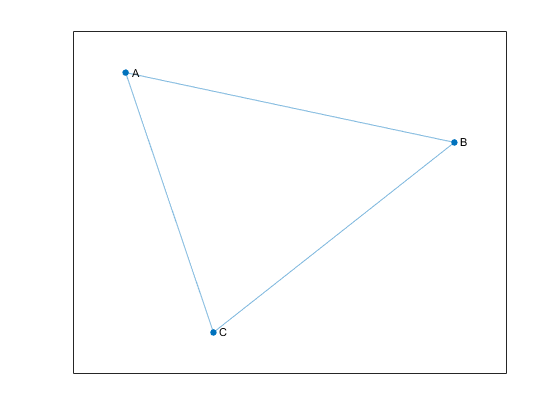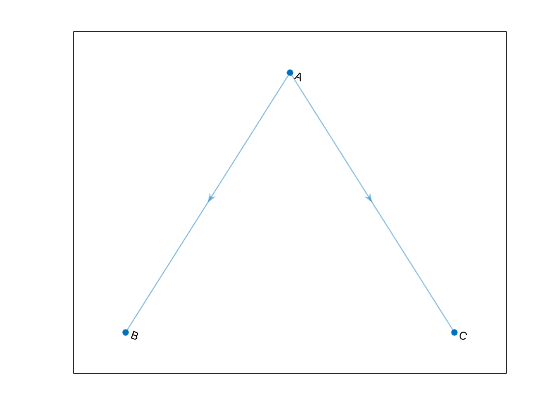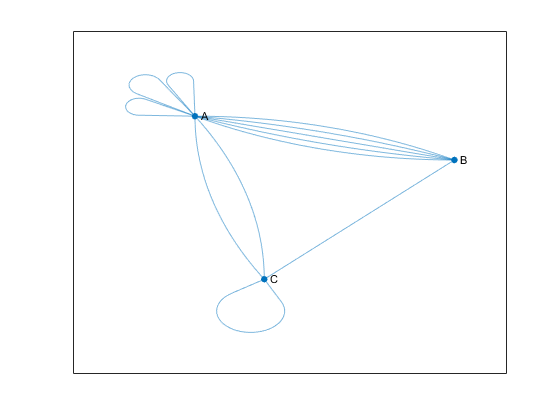## 有向图和无向图

### 什么是图？

• 节点是与对象对应的顶点。

• 是对象之间的连接。

• 图的边有时会有权重，表示节点之间的每个连接的强度（或一些其他属性）。

• 网页链接 - 图节点代表网页，边表示网页之间的超链接。

• 机场 - 图节点代表机场，边表示机场之间的航班。

• 无向图的边没有方向。这些边指示双向关系，因为每条边都可以在两个方向上穿过。下图显示了一个包含三个节点和三条边的简单无向图。• 有向图的边带有方向。这些边指示单向关系，因为每条边只能在单个方向上穿过。下图显示了一个包含三个节点和两条边的简单有向图。#### 自环和多重图

• 节点 A 有三个自环。

• 节点 A 和 B 之间有五条边。

• 节点 A 和 C 之间有两条边。### 创建图

#### 邻接矩阵

• 当您使用 `graph` 创建无向图时，邻接矩阵必须对称。但在实践中，为避免重复，这些矩阵通常为三角形。要仅使用邻接矩阵的上三角或下三角构建无向图，请使用 `graph(A,'upper')``graph(A,'lower')`

• 当您使用 `digraph` 创建有向图时，邻接矩阵不需要对称。

• 对于大型图，邻接矩阵包含许多零并且通常为稀疏矩阵。

• 您不能从邻接矩阵创建多重图。`$\left(\begin{array}{ccc}0& 1& 2\\ 1& 0& 3\\ 2& 3& 0\end{array}\right)\text{\hspace{0.17em}}\text{\hspace{0.17em}}.$`

```A = [0 1 2; 1 0 3; 2 3 0]; node_names = {'A','B','C'}; G = graph(A,node_names)```
```G = graph with properties: Edges: [3×2 table] Nodes: [3×1 table]```

#### 边列表```source_nodes = {'A','A','B'}; target_nodes = {'B','C','C'}; edge_weights = [1 2 3]; G = graph(source_nodes, target_nodes, edge_weights);```

`graph``digraph` 都允许使用边列表构造简单图或多重图。构建图 `G` 后，可以使用命令 `G.Edges` 查看边（及其属性）。这些边在 `G.Edges` 中的顺序首先按源节点（第一列）排列，其次按目标节点（第二列）排列。对于无向图，索引较小的节点列为源节点，索引较大的节点列为目标节点。

### 图节点 ID

```A = [0 1 1 0; 1 0 1 0; 1 1 0 1; 0 0 1 0]; G = graph(A,{'a','b','c','d'}); p = shortestpath(G,1,4) ```
```p = 1 3 4 ```

`p1 = shortestpath(G,'a','d')`
```p1 = 1×3 cell array {'a'} {'c'} {'d'}```

### 修改或查询现有图

 `addedge` 在图中添加一条或多条边 `rmedge` 从图中删除一条或多条边 `addnode` 在图中添加一个或多个节点 `rmnode` 从图中删除一个或多个节点 `findnode` 查找图中的特定节点 `findedge` 查找图中的特定边 `numnodes` 计算图中的节点数 `numedges` 计算图中的边数 `edgecount` 指定的节点之间的边数 `flipedge` 反转有向图边的方向 `reordernodes` 置换图中的节点顺序 `subgraph` 提取子图